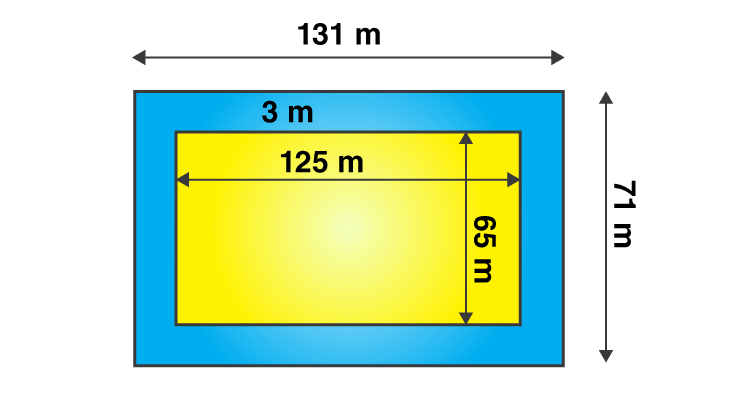# A 3 m wide path runs outside and around a rectangular park of length 125 m and breadth 65 m. Find the area of the path.Given

From the given data we get to know that

Length of the park (L) = 125 m

Breadth of the park (B) = 65 m

Find out

We have to determine the area of the path.

Solution

Area of the park = length × breadth

= 125 × 65

= 8125 m2

From the figure,

The new length and breadth of the park when the path is included is 131 m and 71 m respectively.

A new area of the park = 131 × 71

= 9301 m2

The area of path = New area of the park including path – Area of the park

= 9301 – 8125

= 1176 m2

Are of the path=1176 m2(0)(0)